Algebra Tiles
Base Ten Blocks
Color Tiles
Cuisenaire Rods
Fraction Circles
Fraction Tiles
Geoboard
Hundred Board
Linking Cubes
Number Lines
Pattern Blocks
Place Value Disks
Rekenreks
Two-Color Counters
XY Coordinate Board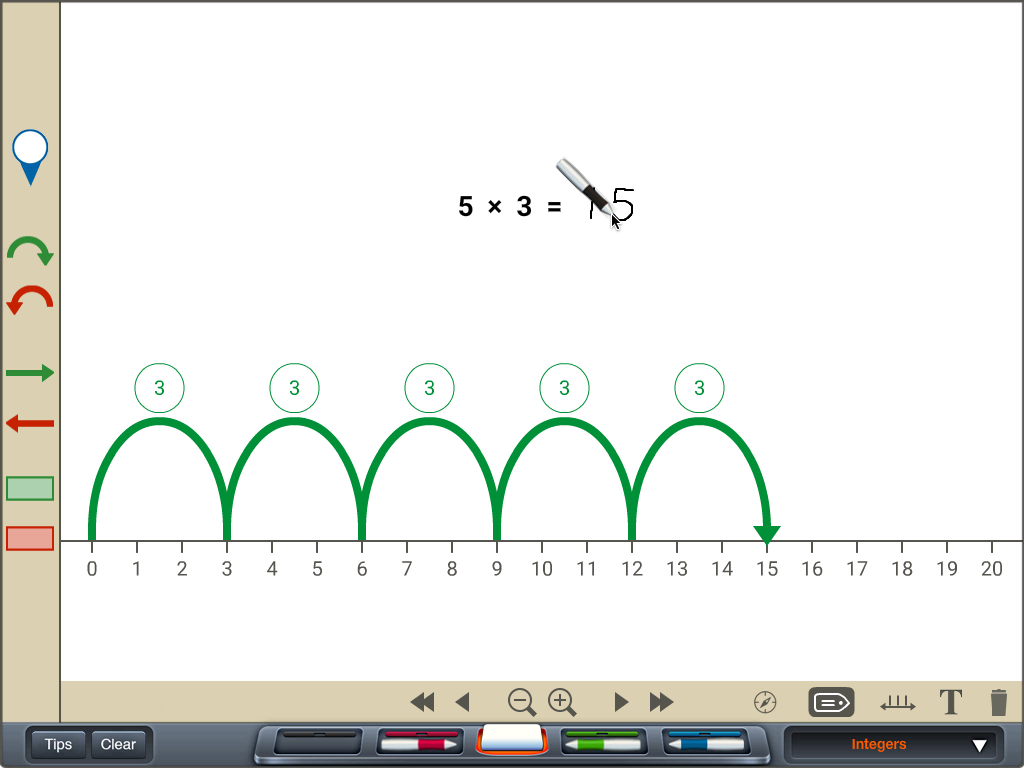5 Ways to MultiplyAdding FractionsAddition as Count and Measure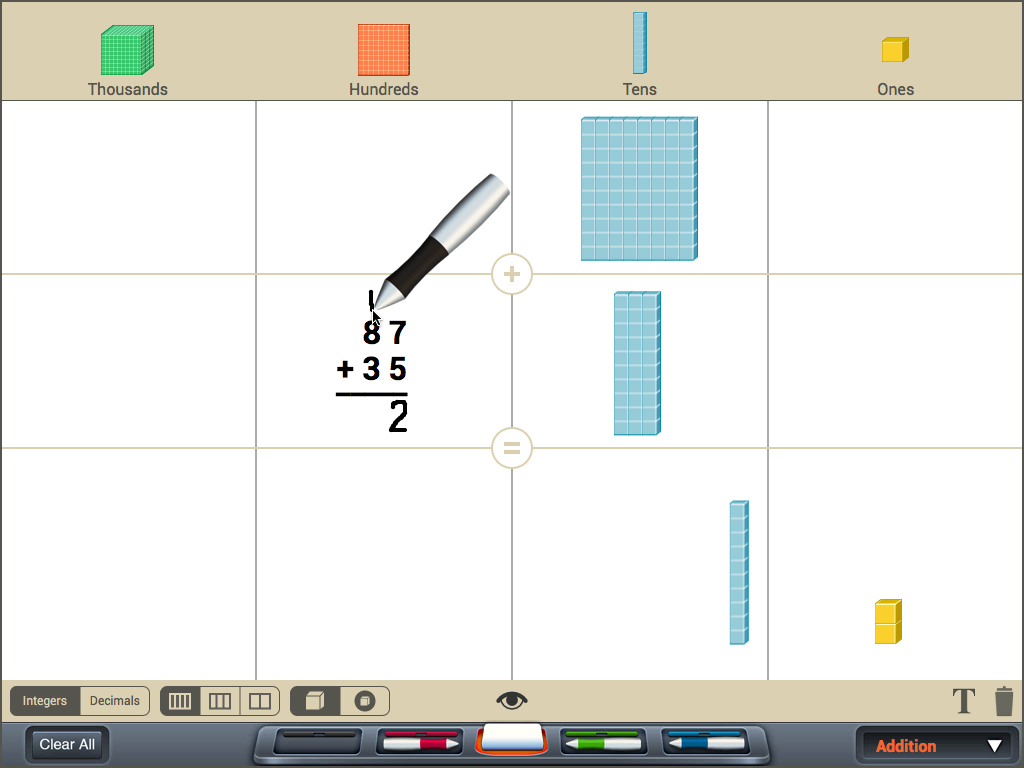Addition with Regrouping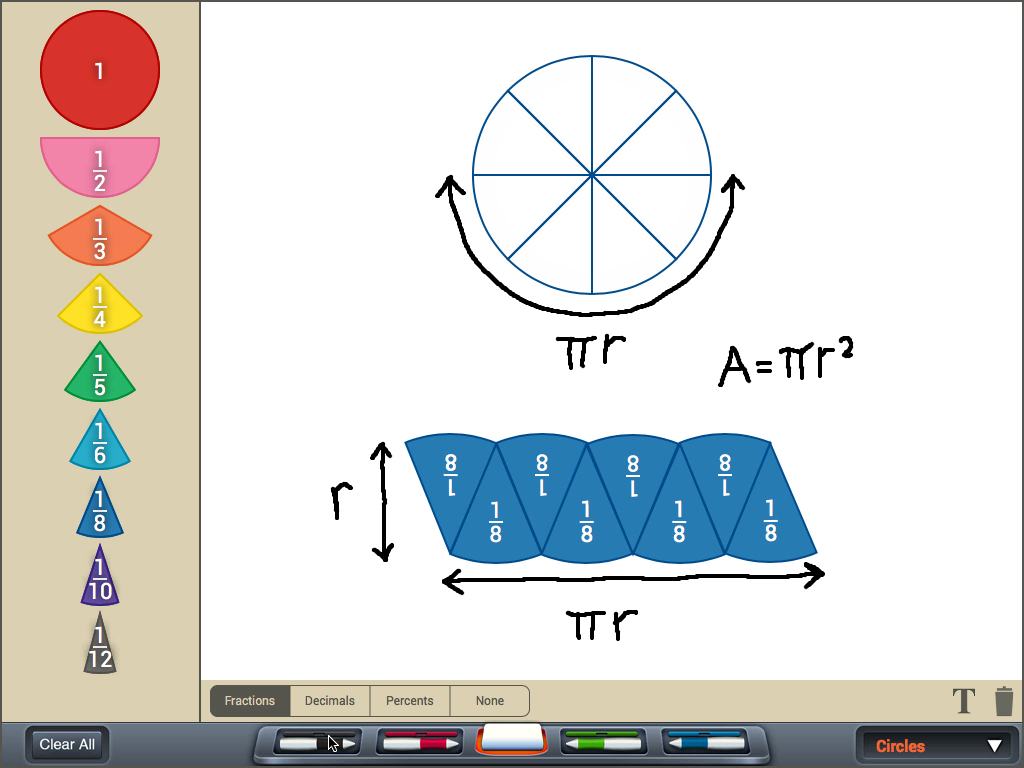Area of a Circle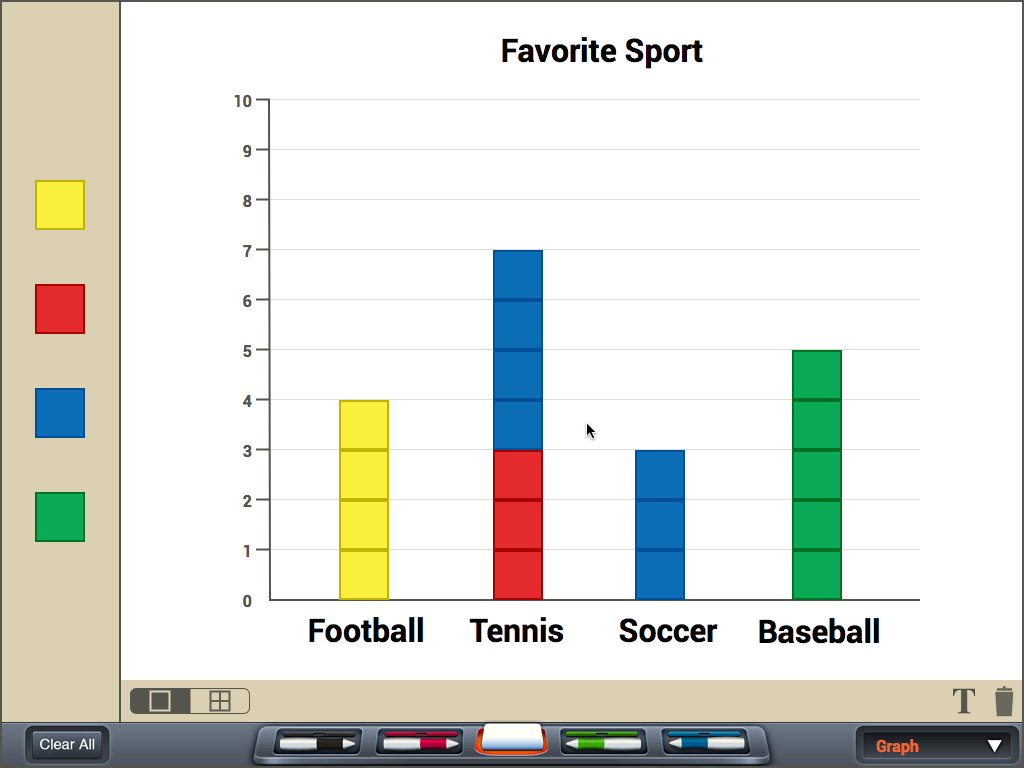Bar Graph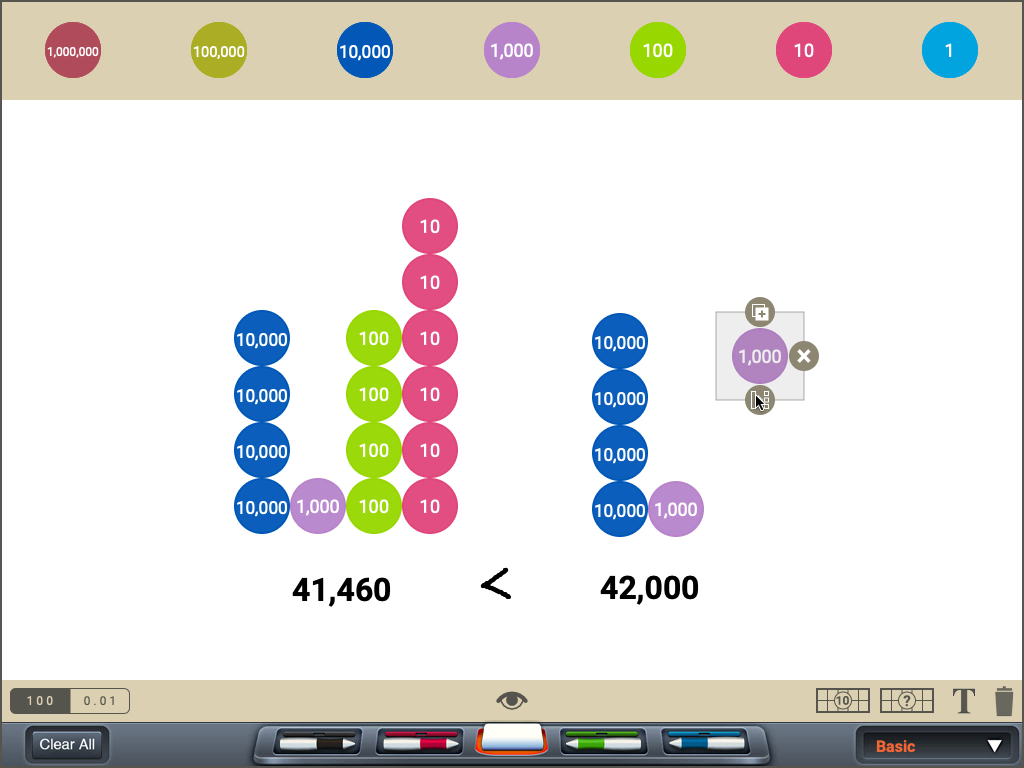Comparing Multi-Digit NumbersCounting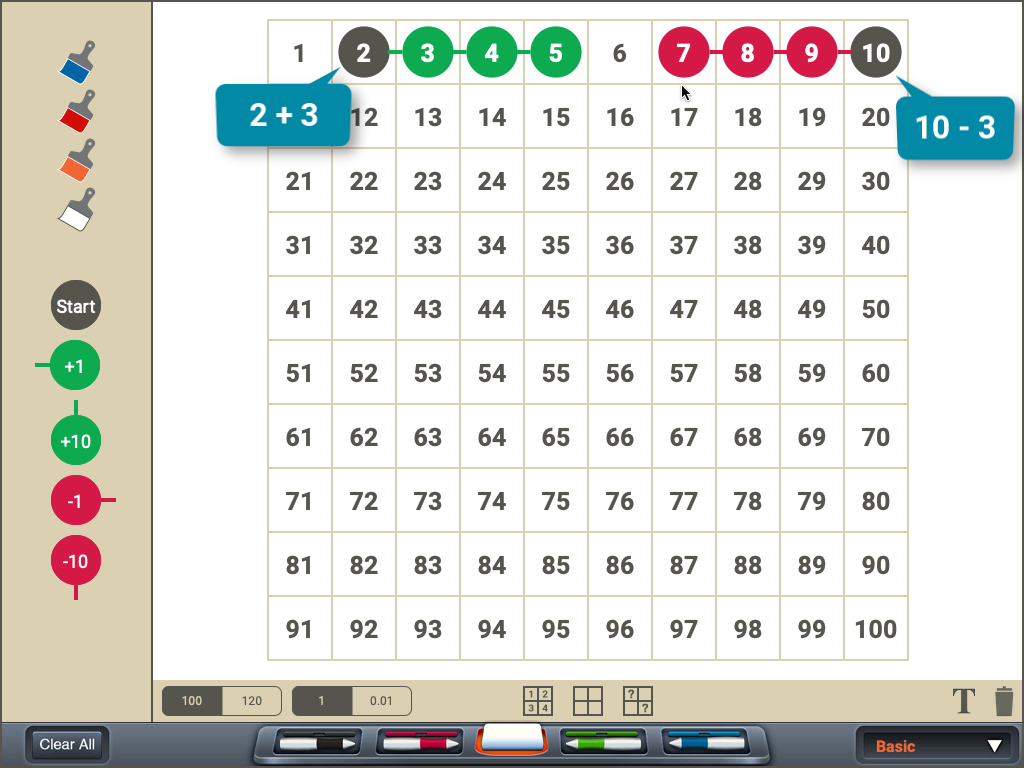Counting On and Back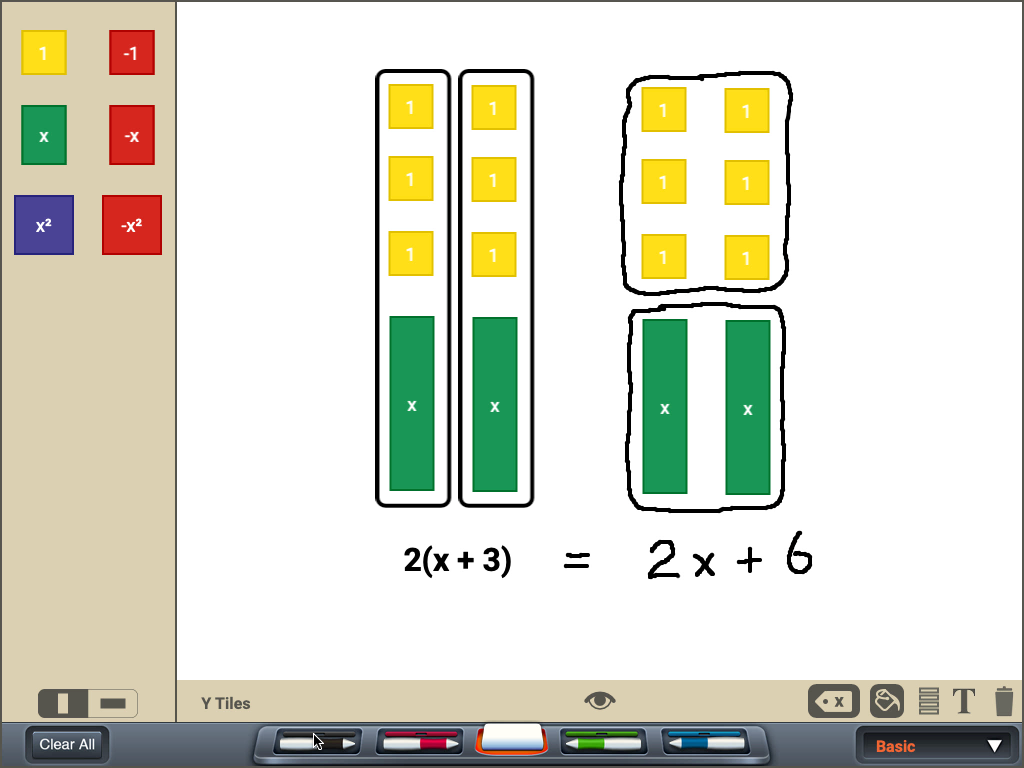Distributive Property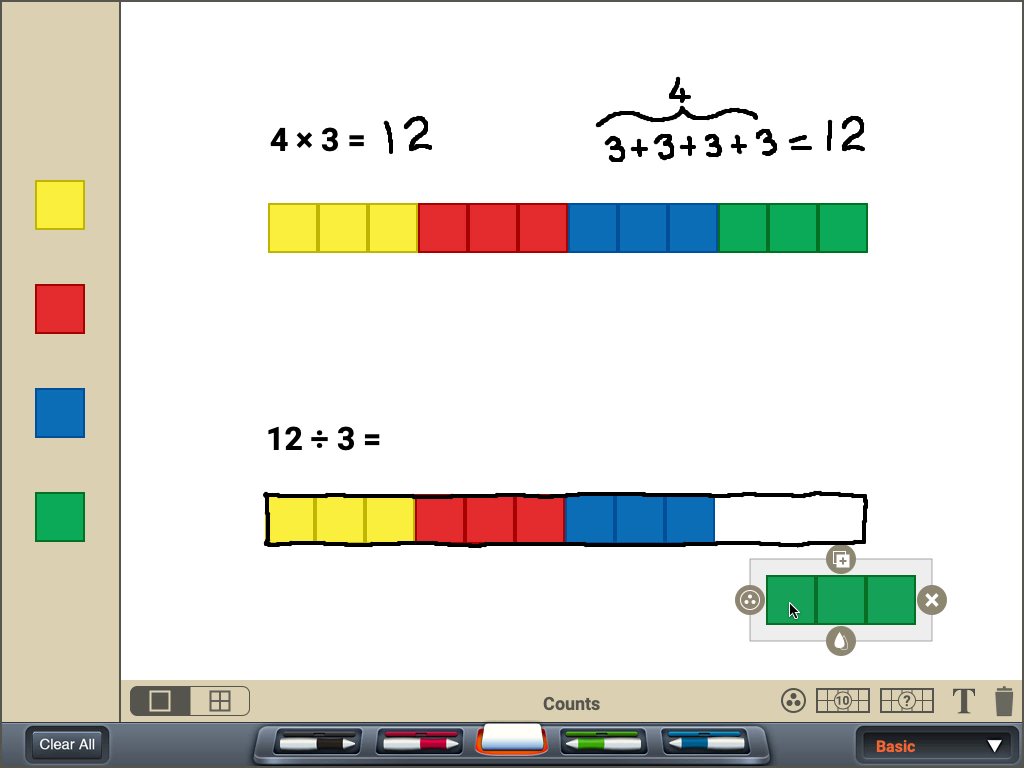Division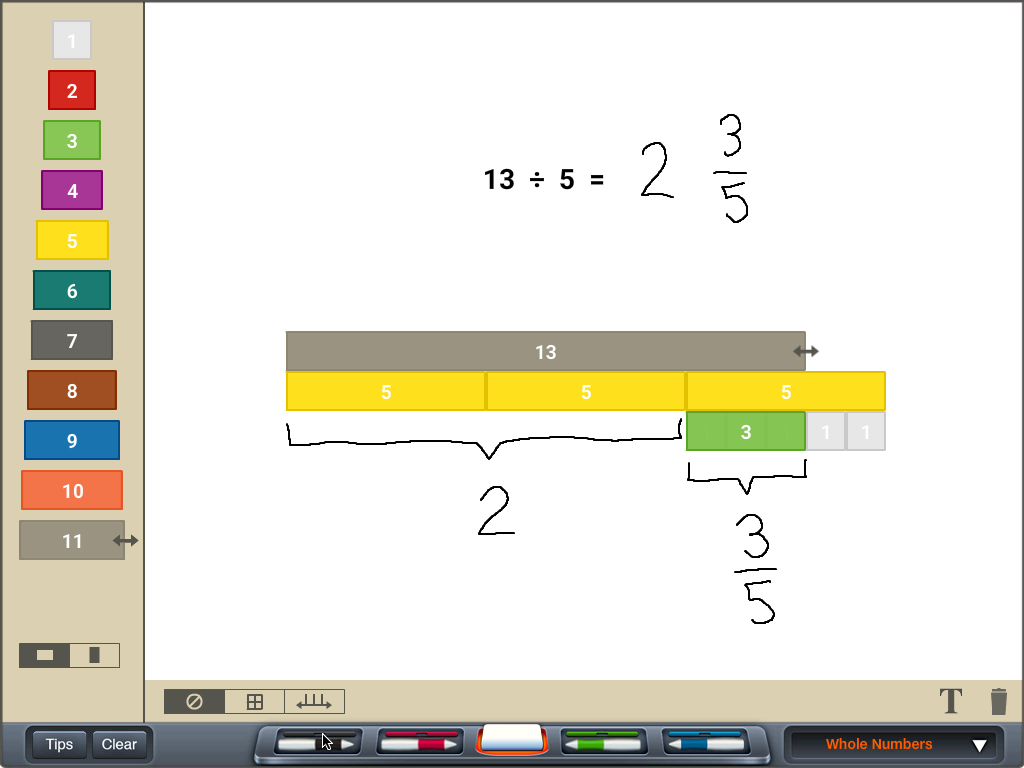Division with a RemainderDivision with Unit Fractions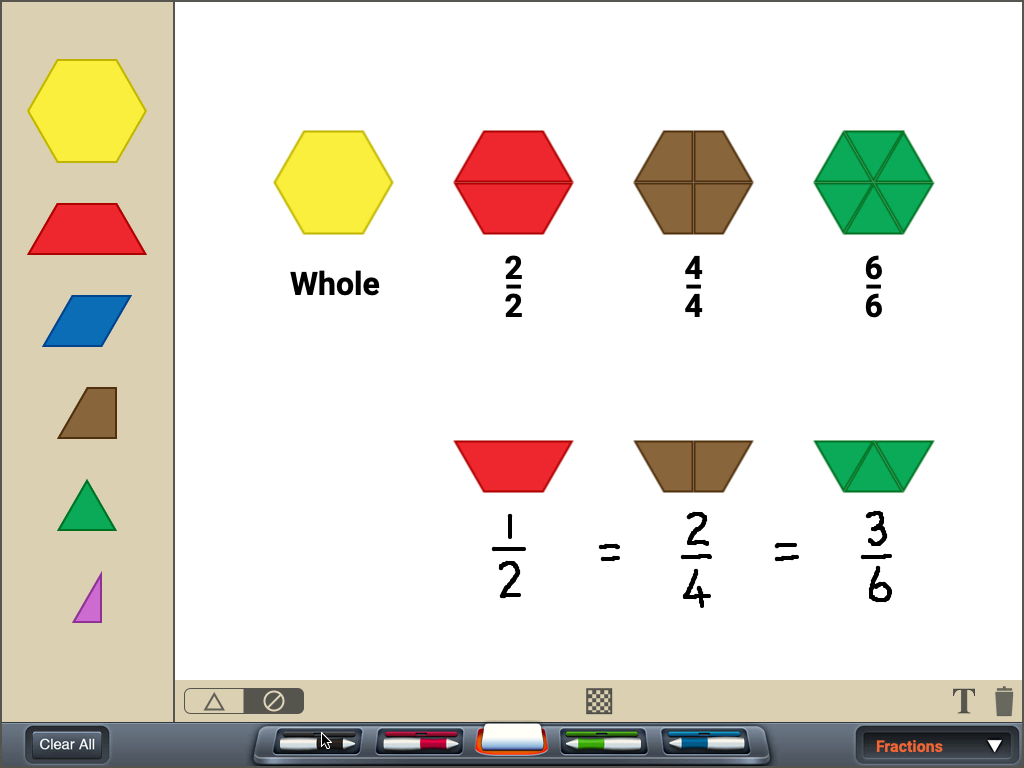Equivalent FractionsEquivalent RatiosEstimating Area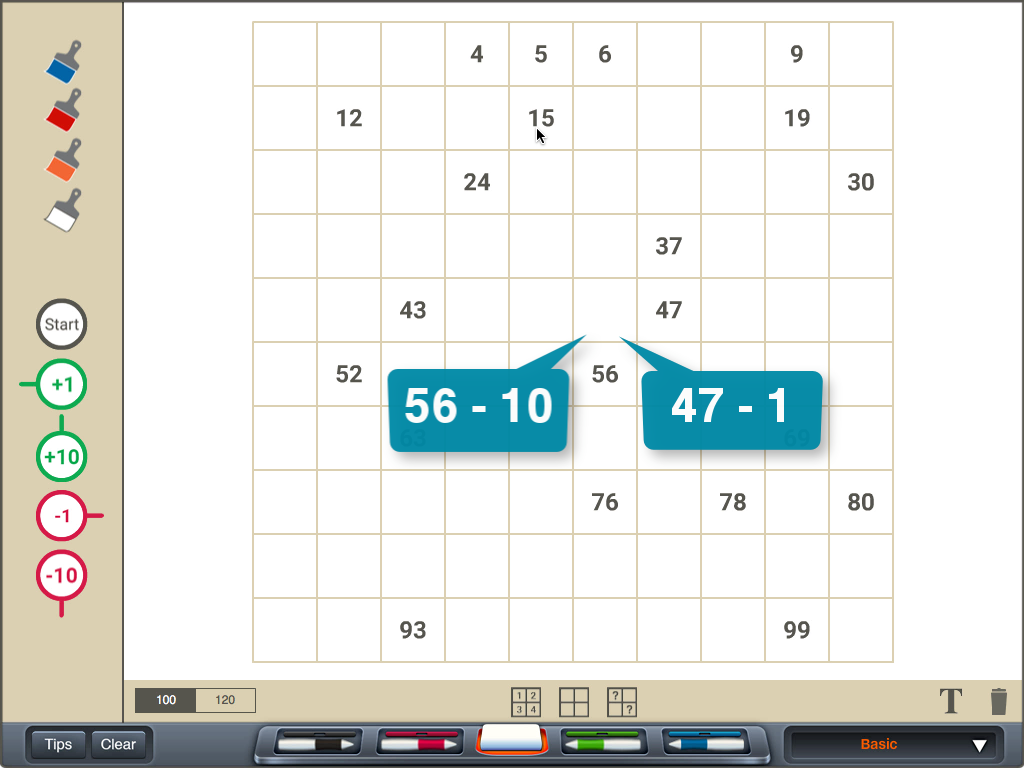Finding Missing NumbersImproper Fractions to Mixed Numbers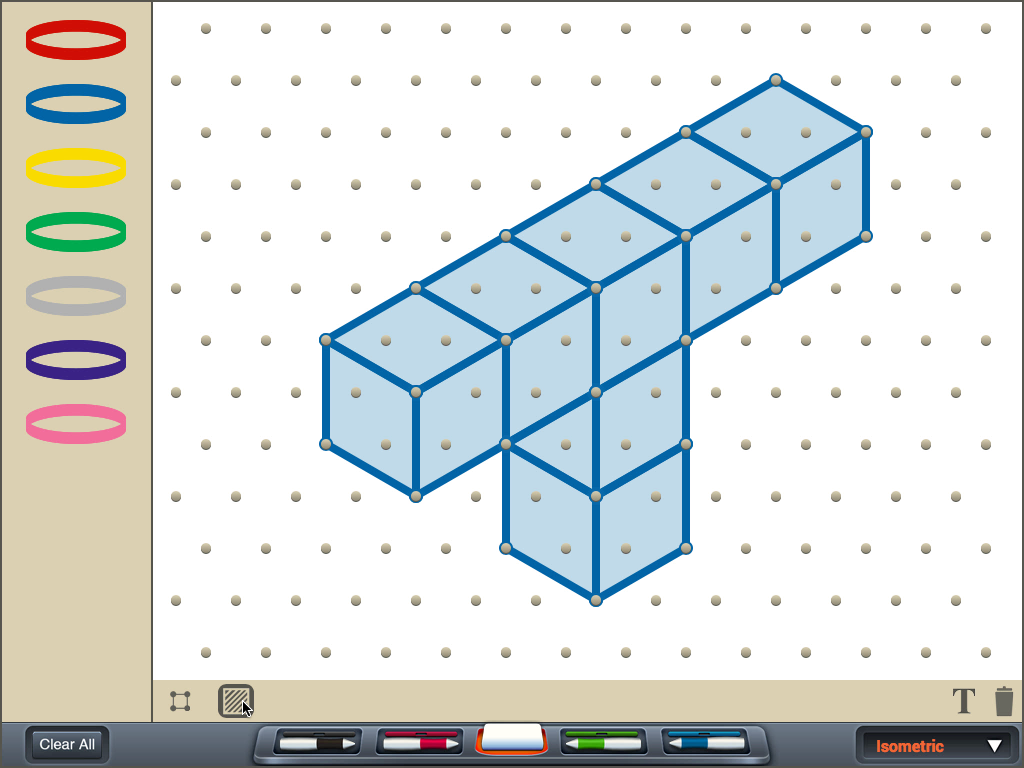Isometric DrawingsMany Ways to Show 213Mixed Numbers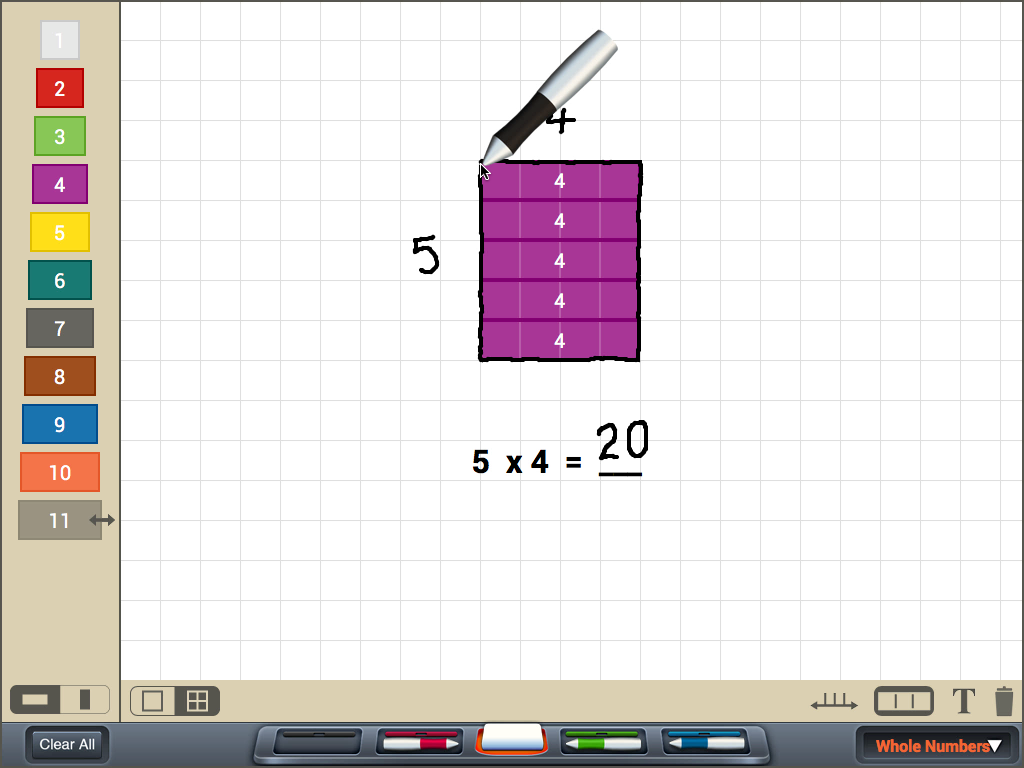Multiplication ModelsMultiplying by Multiple of 10Multiplying Integers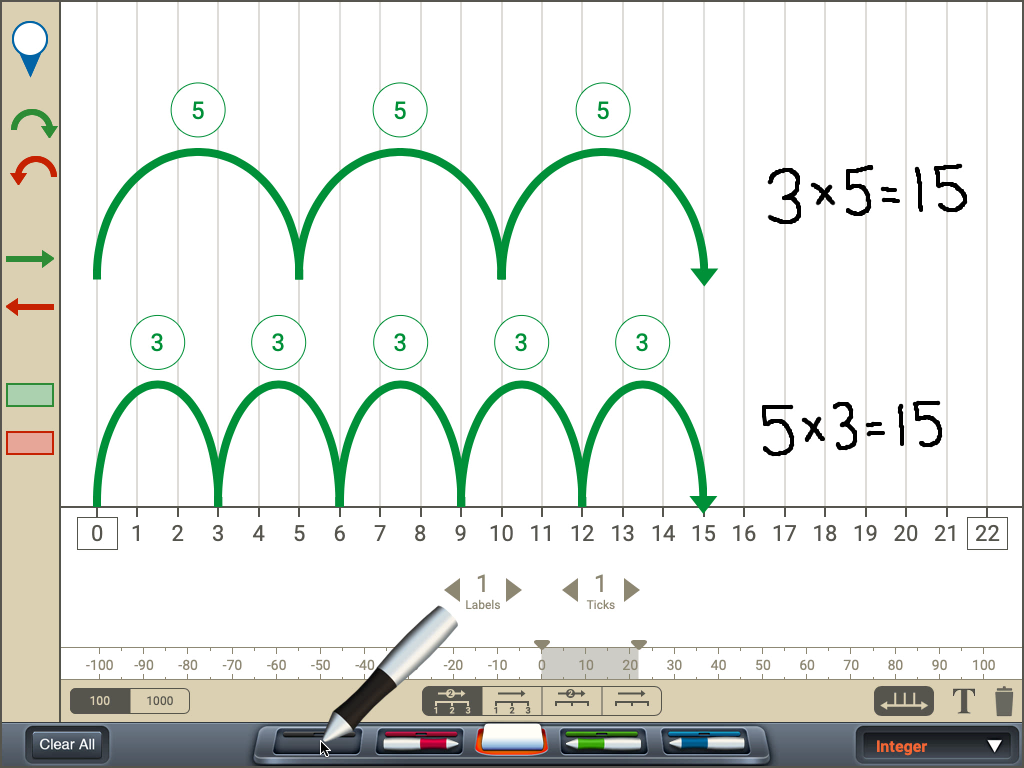Multiplying Whole Numbers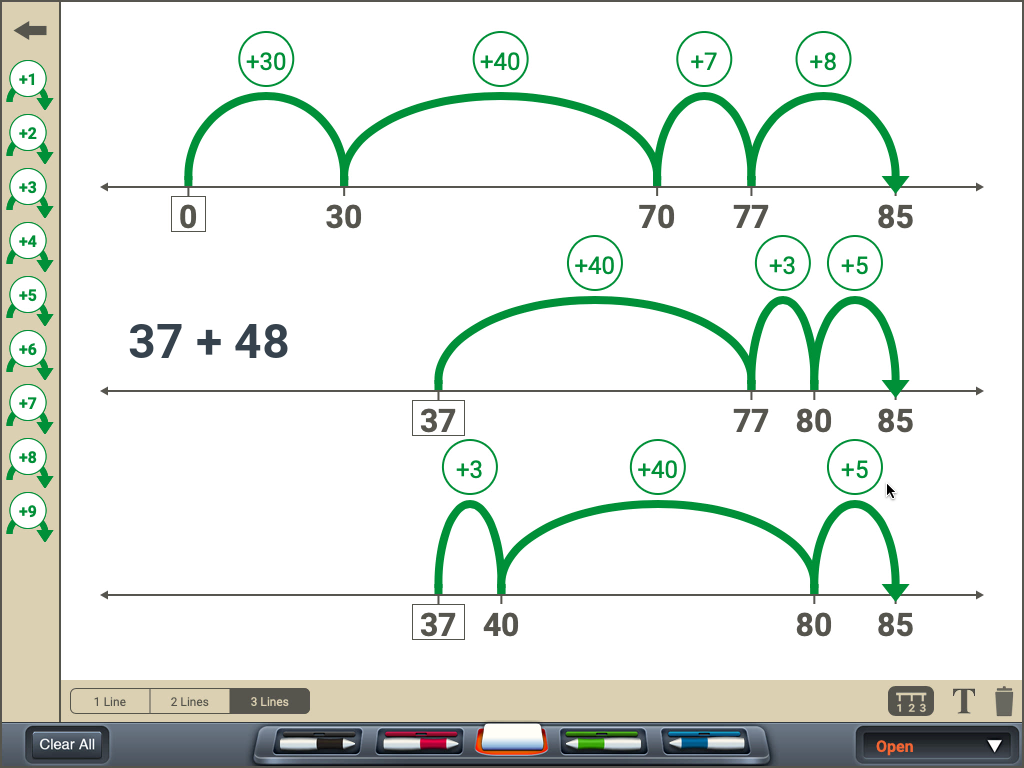Open Number Line AdditionPartitioning Rectangles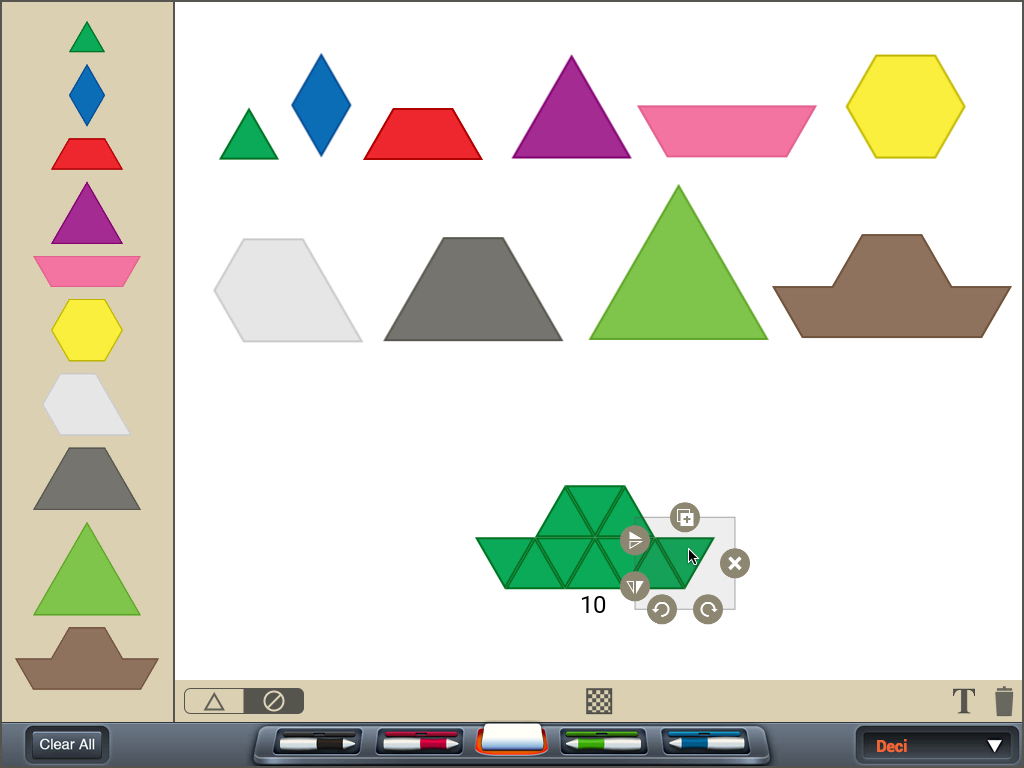Pattern Blocks in Base-10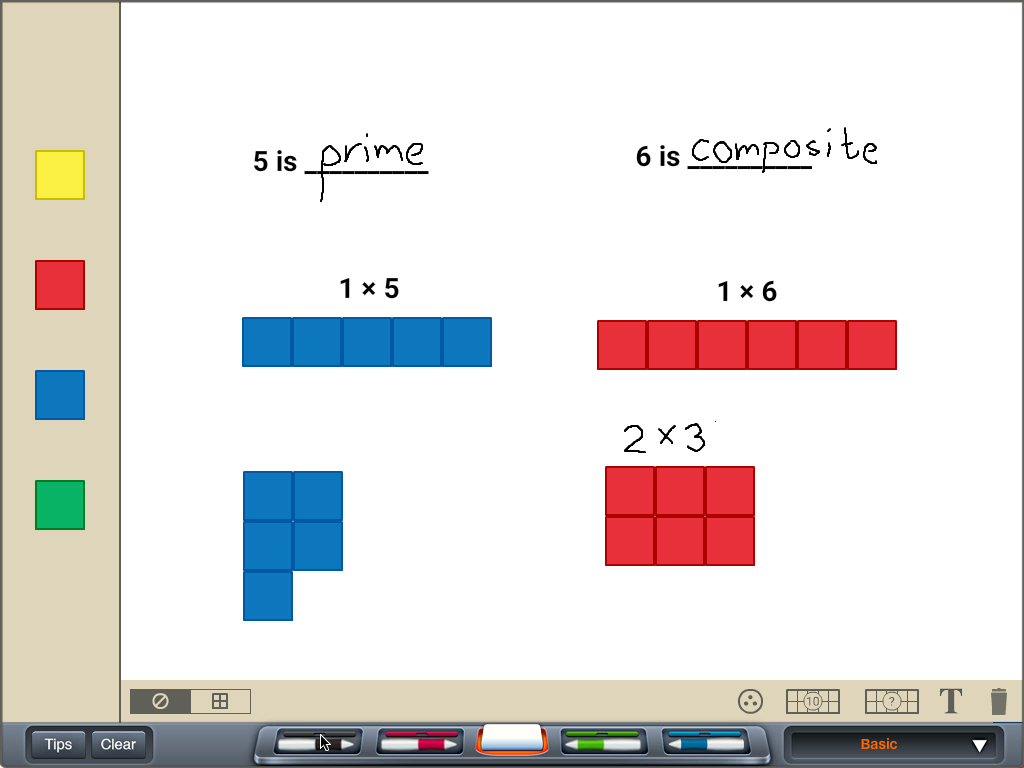Prime and Composite Numbers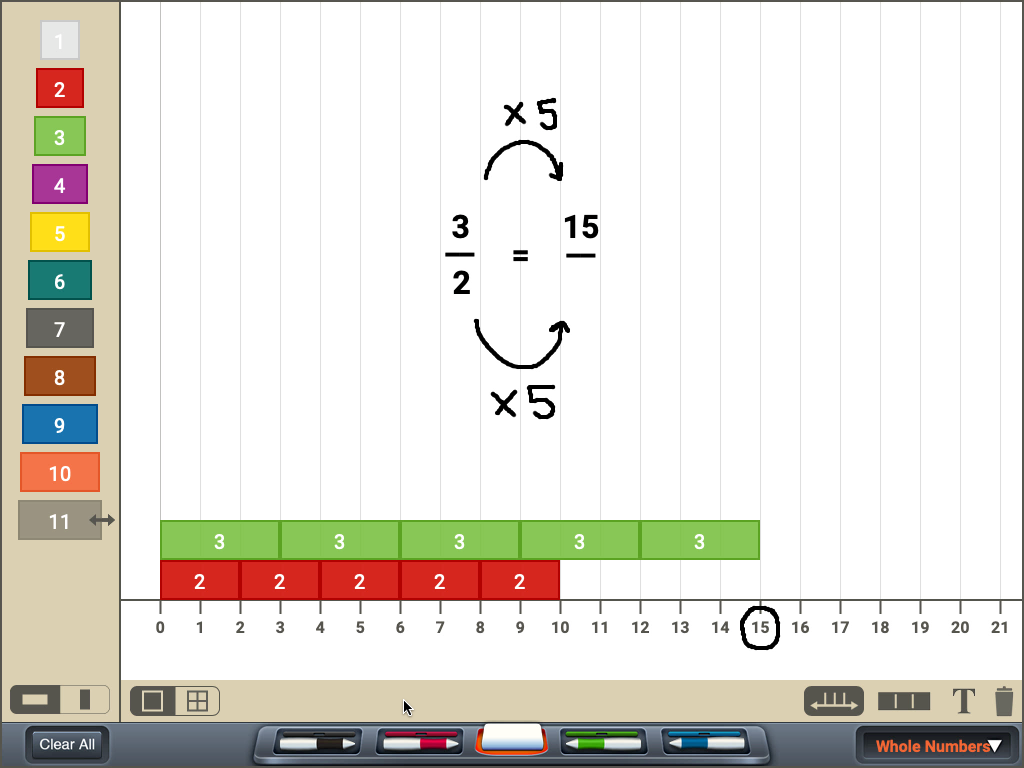Proportions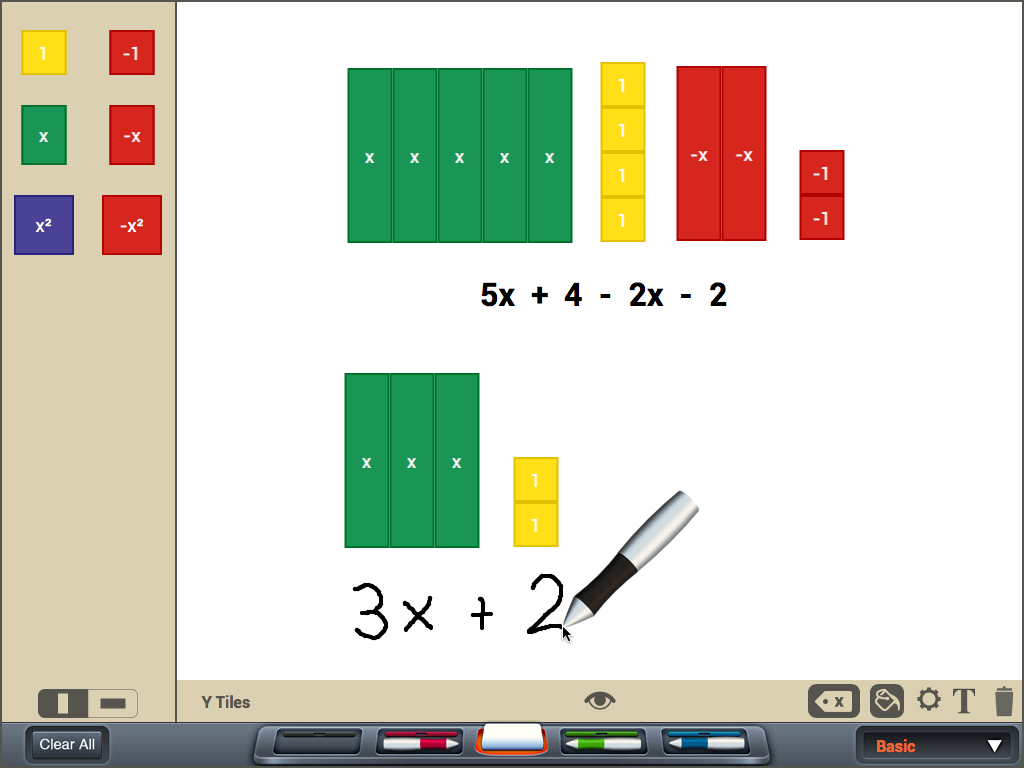Simplifying Expressions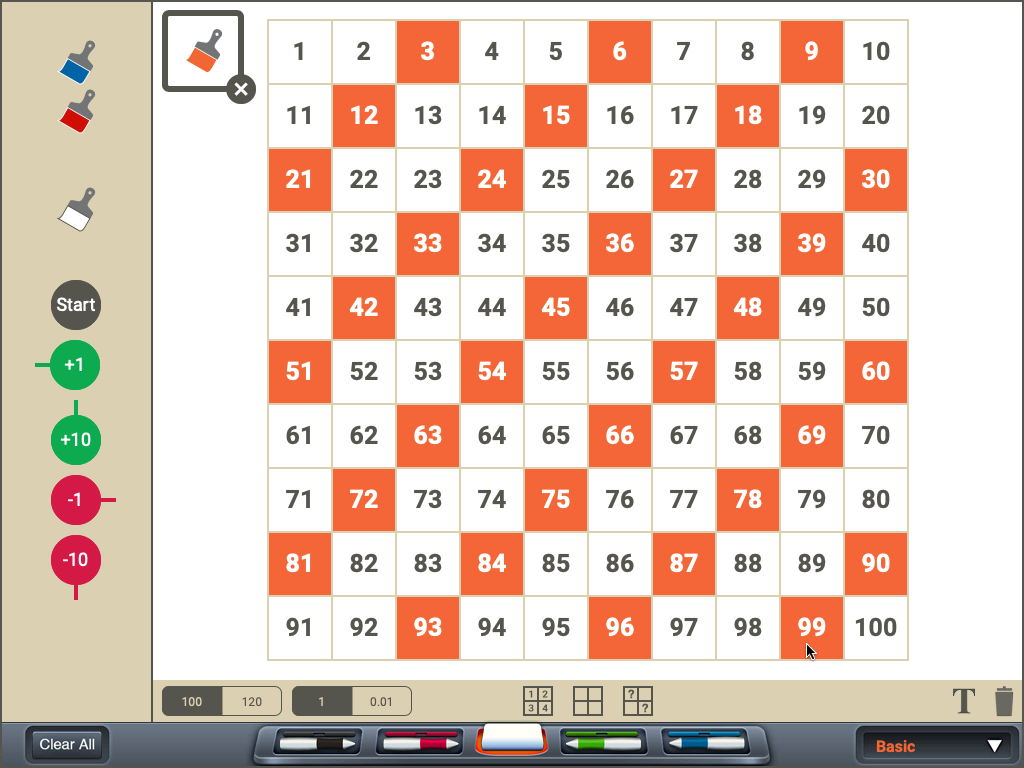Skip Counting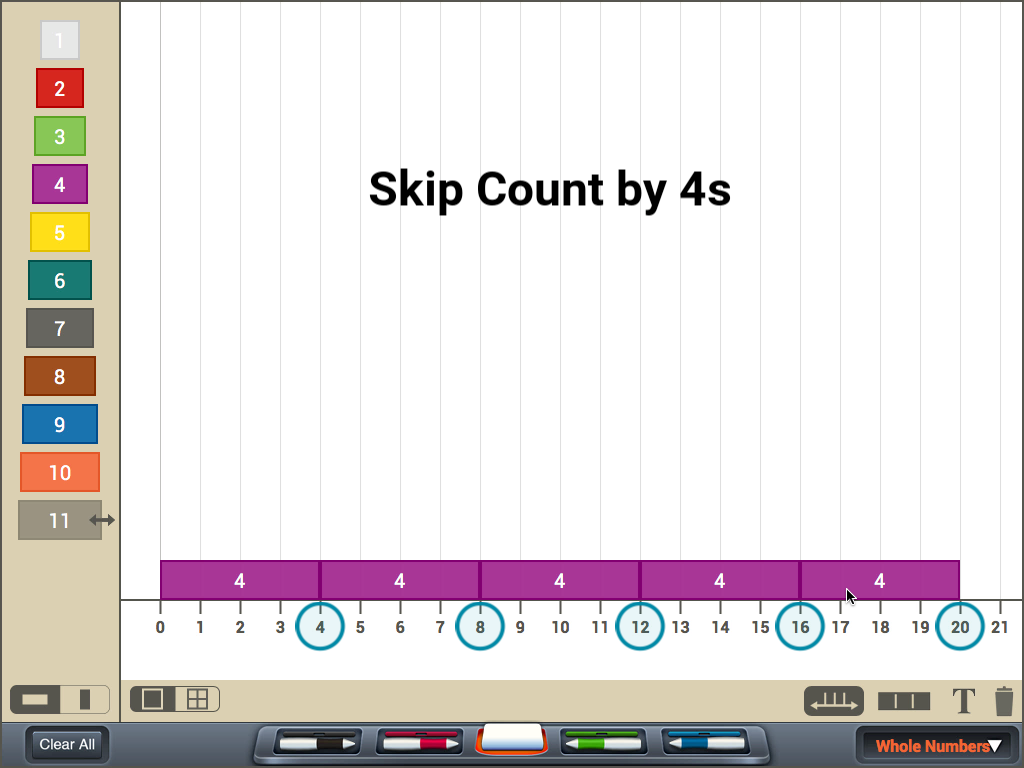Skip Counting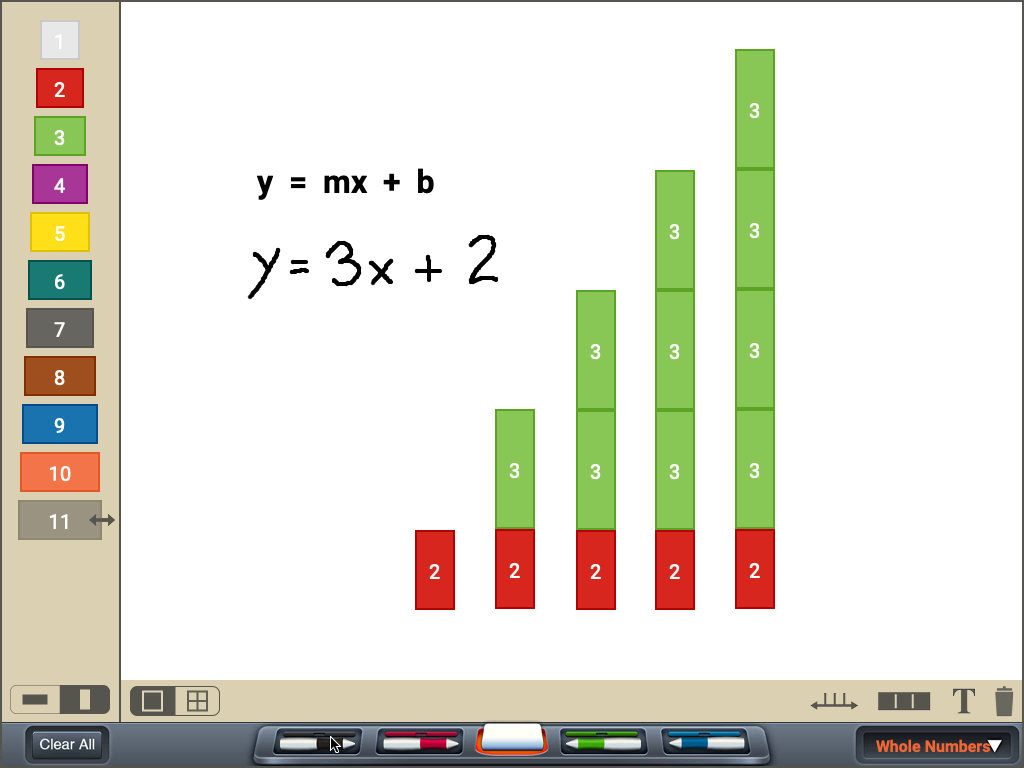Slope Intercept Form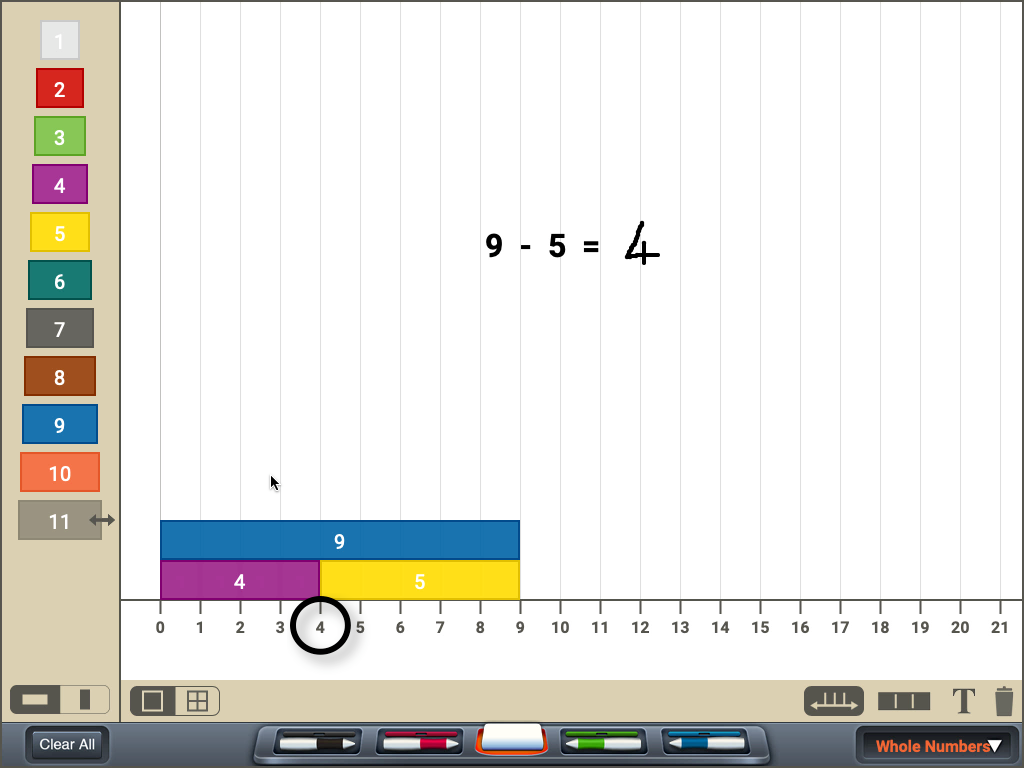SubtractingSubtracting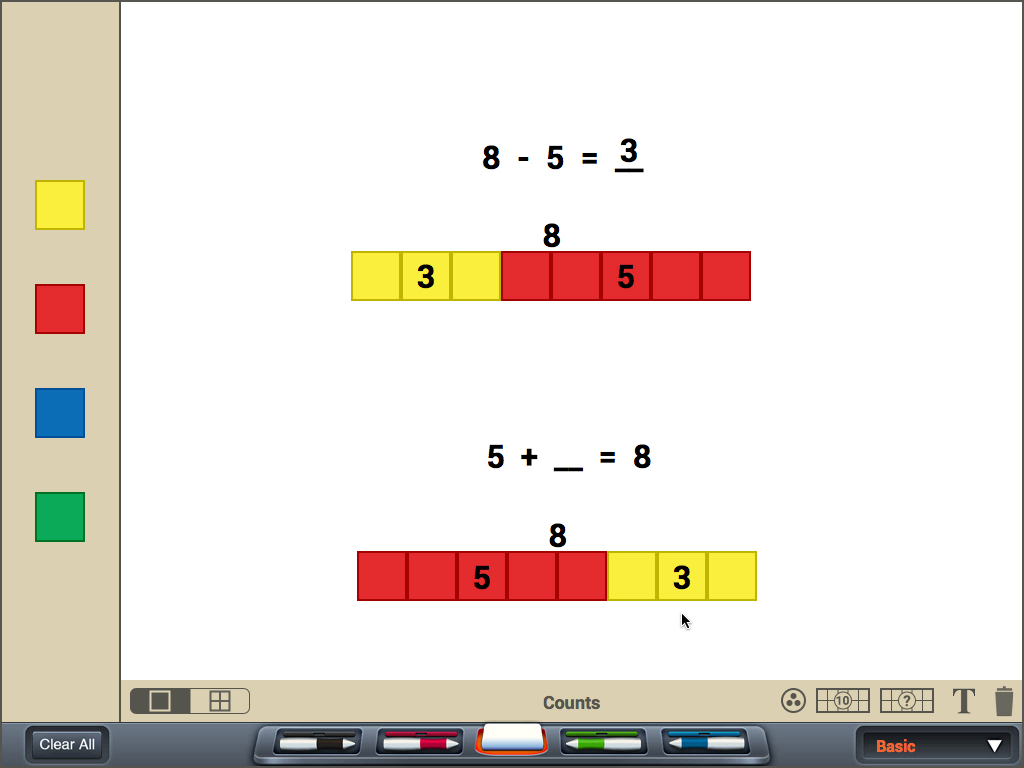Subtracting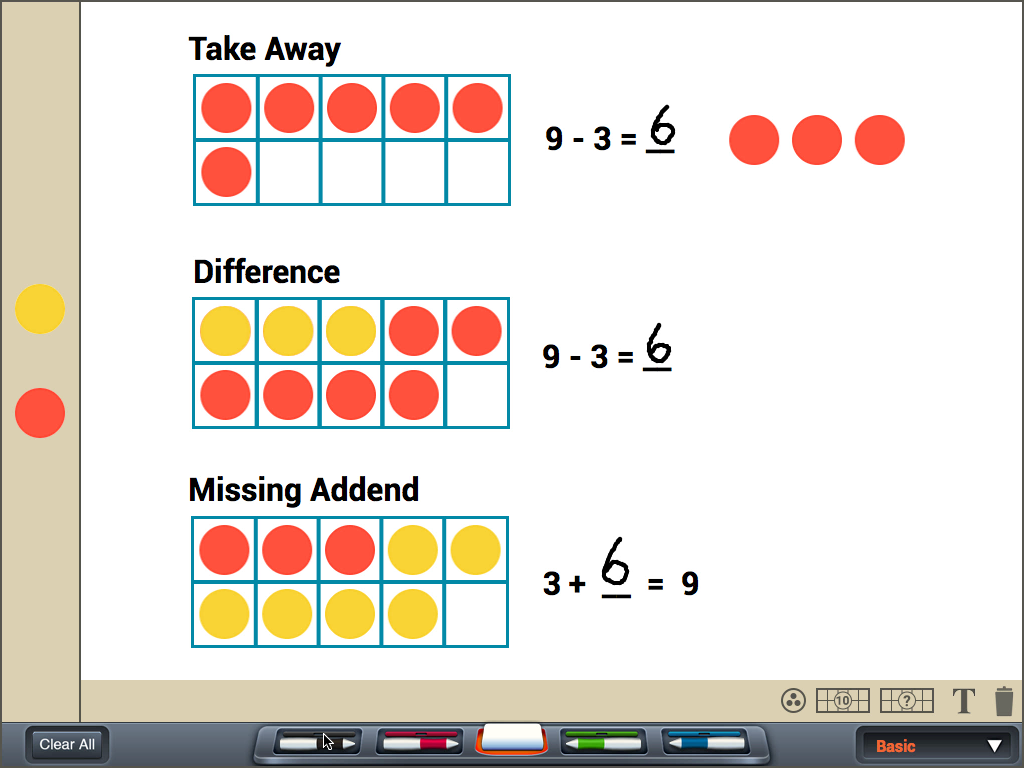Subtraction Strategies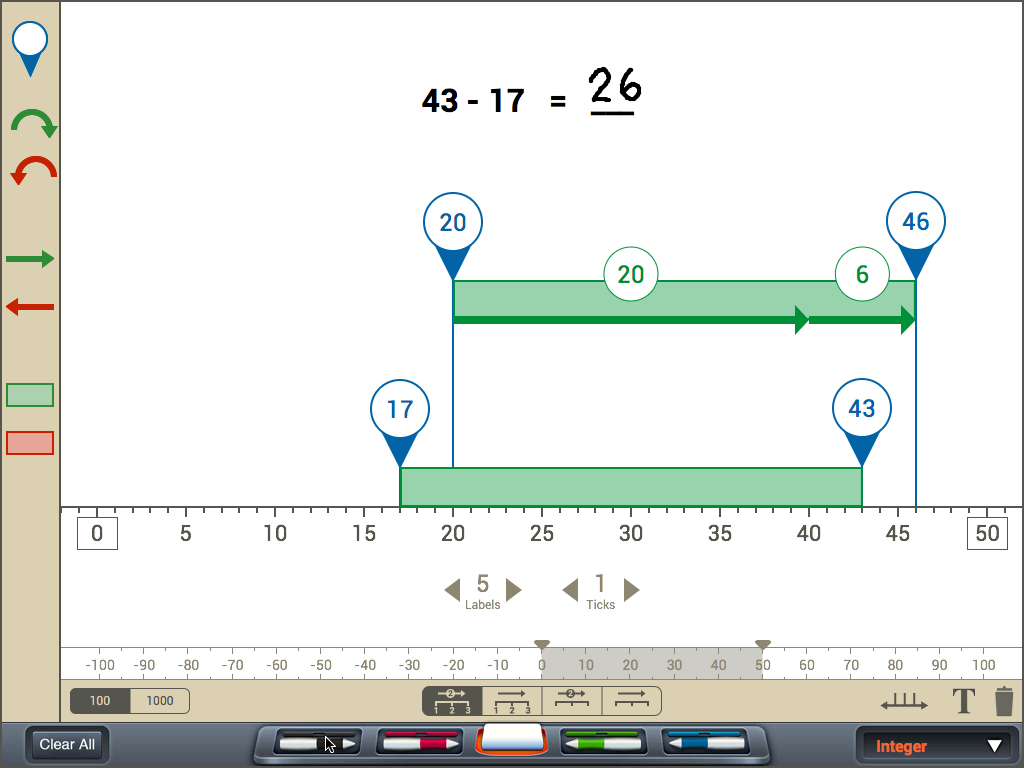Subtraction StrategyTens Frames Addition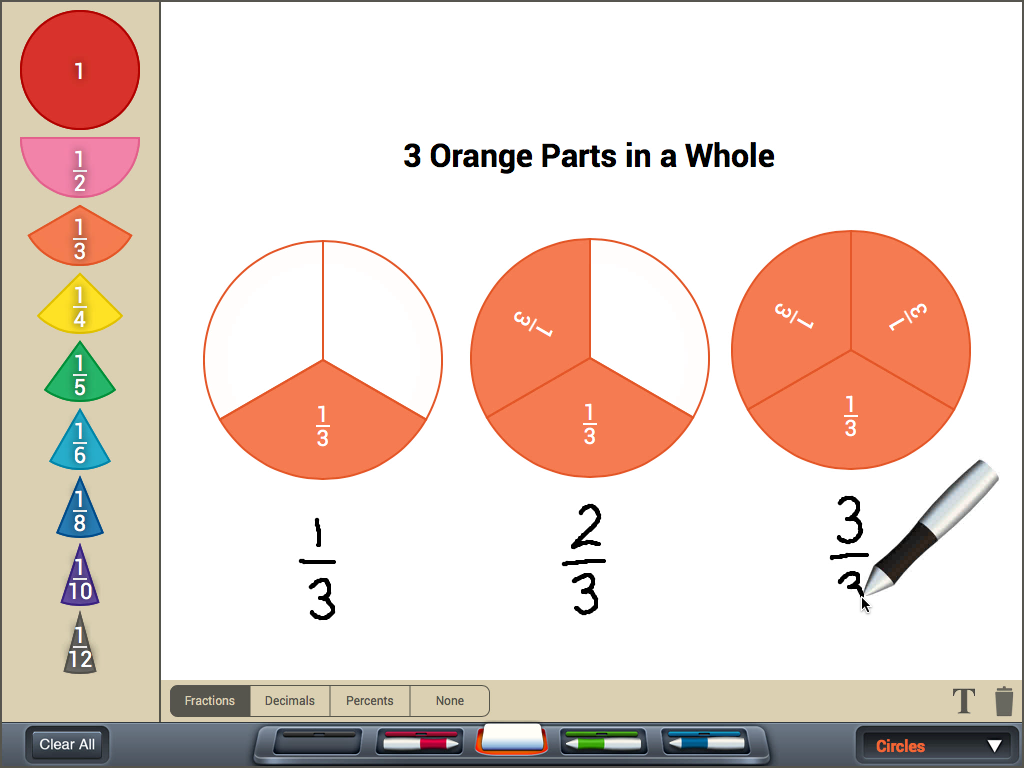Understanding Fractions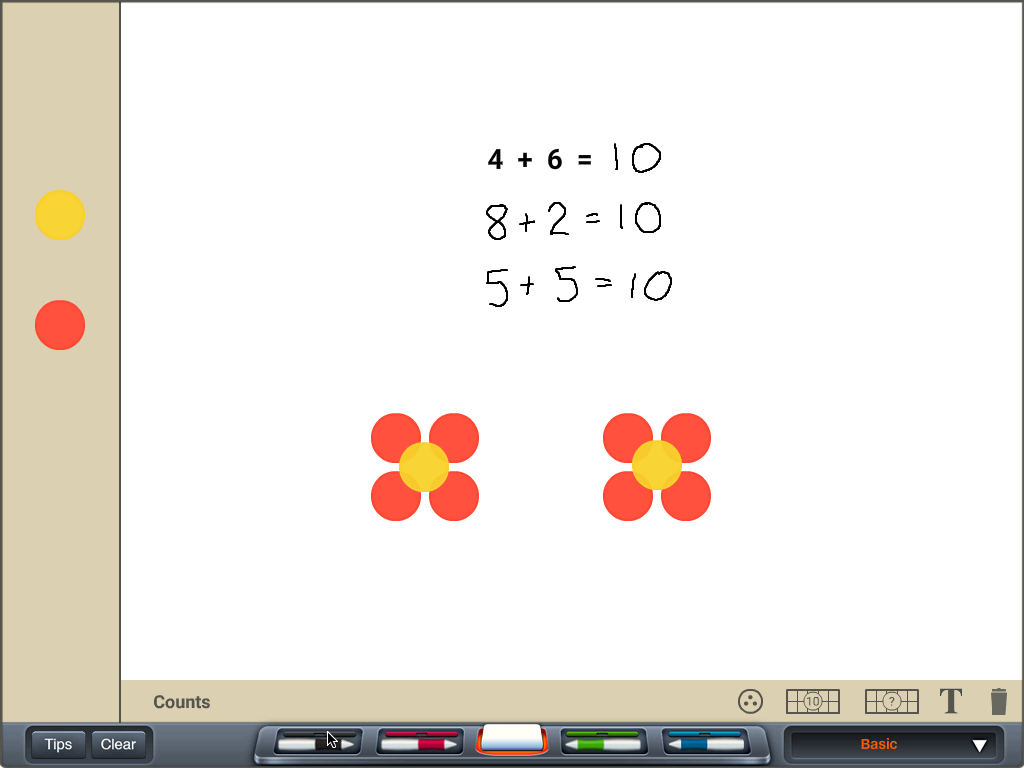Ways to Make a Number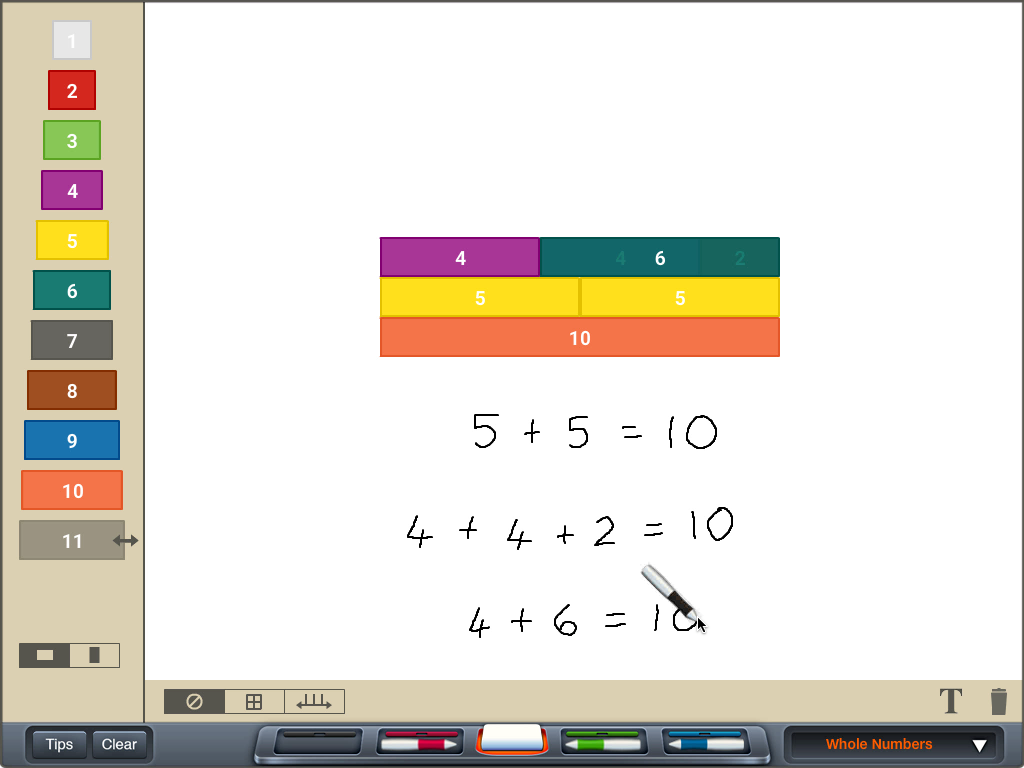What is In a Number